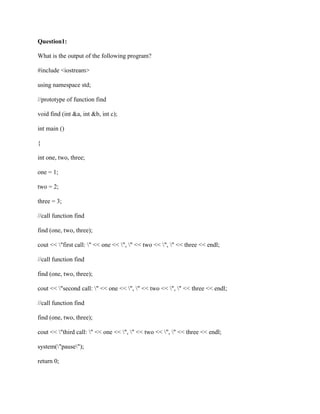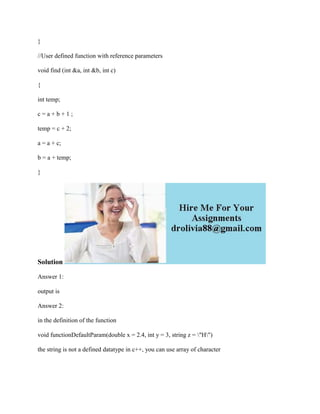Ce diaporama a bien été signalé.
Le téléchargement de votre SlideShare est en cours. ×

# Question1- What is the output of the following program- #include -iost.docx

Publicité
Publicité
Publicité
Publicité
Publicité
Publicité
Publicité
Publicité
Publicité
Publicité
PublicitéChargement dans…3
×

## Consultez-les par la suite

1 sur 3 Publicité

# Question1- What is the output of the following program- #include -iost.docx

Question1:
What is the output of the following program?
#include <iostream>
using namespace std;
//prototype of function find
void find (int &a, int &b, int c);
int main ()
{
int one, two, three;
one = 1;
two = 2;
three = 3;
//call function find
find (one, two, three);
cout << \"first call: \" << one << \", \" << two << \", \" << three << endl;
//call function find
find (one, two, three);
cout << \"second call: \" << one << \", \" << two << \", \" << three << endl;
//call function find
find (one, two, three);
cout << \"third call: \" << one << \", \" << two << \", \" << three << endl;
system(\"pause\");
return 0;
}
//User defined function with reference parameters
void find (int &a, int &b, int c)
{
int temp;
c = a + b + 1 ;
temp = c + 2;
a = a + c;
b = a + temp;
}
Solution
output is
in the definition of the function
void functionDefaultParam(double x = 2.4, int y = 3, string z = \"H\")
the string is not a defined datatype in c++, you can use array of character
.

Question1:
What is the output of the following program?
#include <iostream>
using namespace std;
//prototype of function find
void find (int &a, int &b, int c);
int main ()
{
int one, two, three;
one = 1;
two = 2;
three = 3;
//call function find
find (one, two, three);
cout << \"first call: \" << one << \", \" << two << \", \" << three << endl;
//call function find
find (one, two, three);
cout << \"second call: \" << one << \", \" << two << \", \" << three << endl;
//call function find
find (one, two, three);
cout << \"third call: \" << one << \", \" << two << \", \" << three << endl;
system(\"pause\");
return 0;
}
//User defined function with reference parameters
void find (int &a, int &b, int c)
{
int temp;
c = a + b + 1 ;
temp = c + 2;
a = a + c;
b = a + temp;
}
Solution
output is